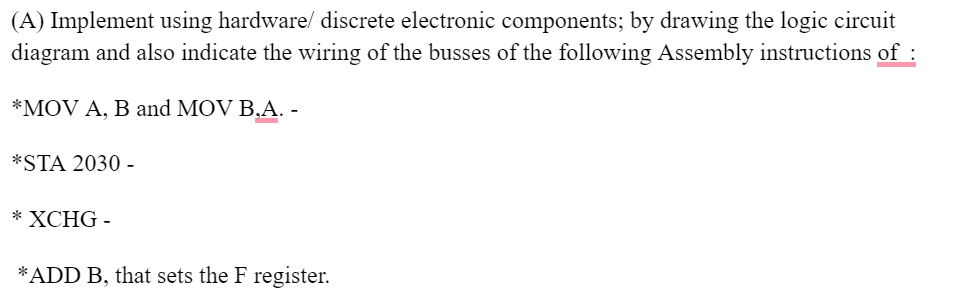# Question (A) Implement using hardware/ discrete electronic components; by drawing the logic circuit diagram and also indicate the wiring of the busses of the following Assembly instructions of : $$* \mathrm{MOV} \mathrm{A}, \mathrm{B}$$ and $$\mathrm{MOV} \mathrm{B}, \mathrm{A}$$. - $$* \operatorname{STA} 2030$$ $$* \mathrm{XCHG}-$$ *ADD B, that sets the $$\mathrm{F}$$ register.28AJRO The Asker · Electrical EngineeringTranscribed Image Text: (A) Implement using hardware/ discrete electronic components; by drawing the logic circuit diagram and also indicate the wiring of the busses of the following Assembly instructions of : $$* \mathrm{MOV} \mathrm{A}, \mathrm{B}$$ and $$\mathrm{MOV} \mathrm{B}, \mathrm{A}$$. - $$* \operatorname{STA} 2030$$ $$* \mathrm{XCHG}-$$ *ADD B, that sets the $$\mathrm{F}$$ register.
More
Transcribed Image Text: (A) Implement using hardware/ discrete electronic components; by drawing the logic circuit diagram and also indicate the wiring of the busses of the following Assembly instructions of : $$* \mathrm{MOV} \mathrm{A}, \mathrm{B}$$ and $$\mathrm{MOV} \mathrm{B}, \mathrm{A}$$. - $$* \operatorname{STA} 2030$$ $$* \mathrm{XCHG}-$$ *ADD B, that sets the $$\mathrm{F}$$ register.